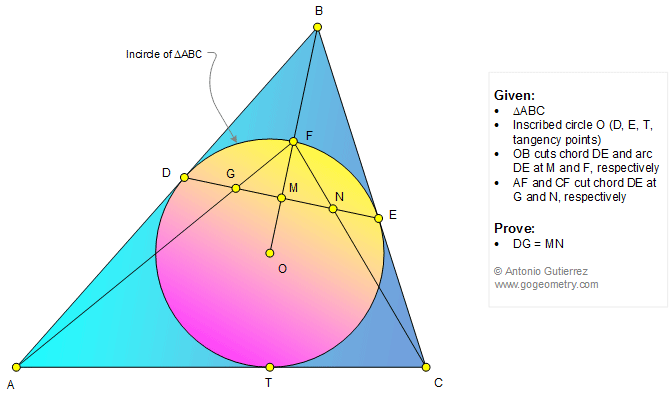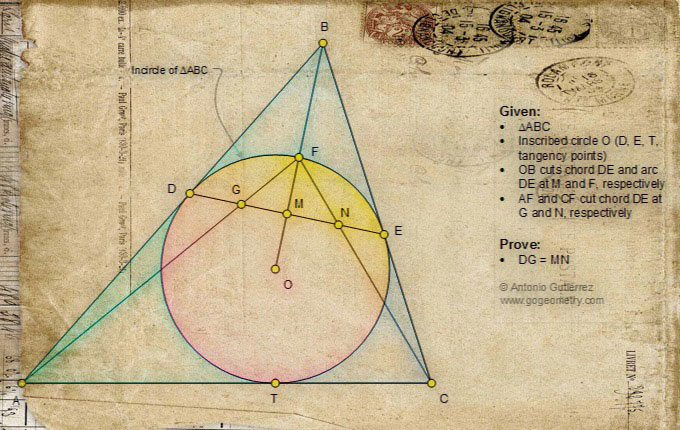#Geometry Problem 1315: Triangle, Incircle, Tangent, Chord, Circle, Congruence. Sketch, Software

< PREVIOUS PROBLEM  |  NEXT PROBLEM >

The figure shows a triangle ABC with the inscribed circle O (D, E, and T are the tangency points). OB cuts chord DE and arc DE at M and F, respectively. AF and CF cut chord DE at G and N, respectively. Prove that DG = MN.## Sketch of problem 1315See also:
Problems
All Problems
Open Problems
Visual Index
1311-1320
Triangle
Circle
Incircle
Tangent line
Congruence
View or Post a solution

## Geometry Problem 1315 on Twitter:

 Home | Geometry | Email